Home » Remote Sensing » Geosynchronous vs Geostationary Orbits

# Geosynchronous vs Geostationary Orbits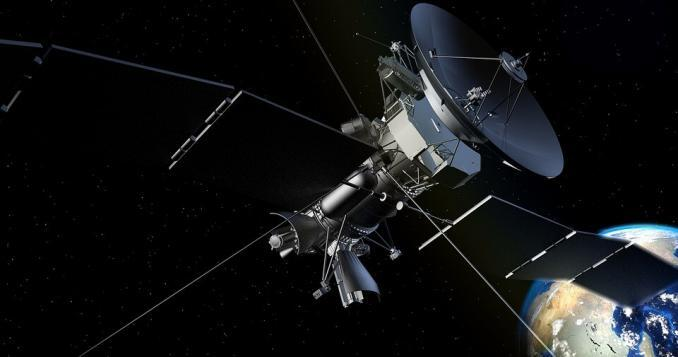### What is the difference between geosynchronous and geostationary orbits?

There’s a sweet spot above the Earth where a satellite can match the same rotation of the Earth.

This special position in high Earth orbit is known as a geosynchronous orbit.

But how is this any different from a geostationary orbit?

Let’s dive into some of the differences between geosynchronous and geostationary orbits.

#### Geosynchronous Orbit

About 35,786 kilometers above the Earth’s surface, satellites are in geostationary orbit. From the center of the Earth, this is approximately 42,164 kilometers. This distance puts it in the high Earth orbit category.

At any inclination, a geosynchronous orbit synchronizes with the rotation of the Earth. More specifically, the time it takes for the Earth to rotate on its axis is 23 hours, 56 minutes, and 4.09 seconds, which is the same as a satellite in a geosynchronous orbit.

If you are an observer on the ground, you would see the satellite as if it’s in a fixed position without movement.

This makes geosynchronous satellites particularly useful for telecommunications and other remote sensing applications.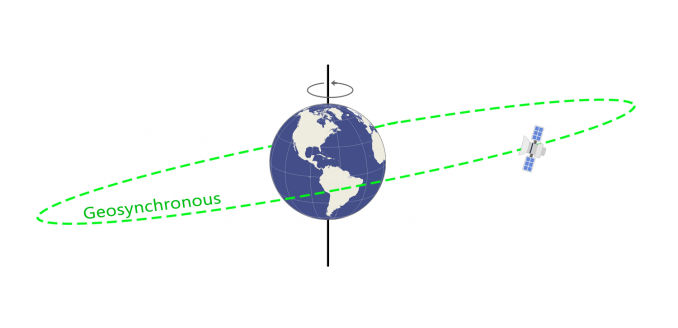#### Geostationary Orbit

While geosynchronous satellites can have any inclination, the key difference to geostationary orbit is the fact that they lie on the same plane as the equator.

Geostationary orbits fall in the same category as geosynchronous orbits, but it’s parked over the equator. This one special quality makes it unique from geosynchronous orbits.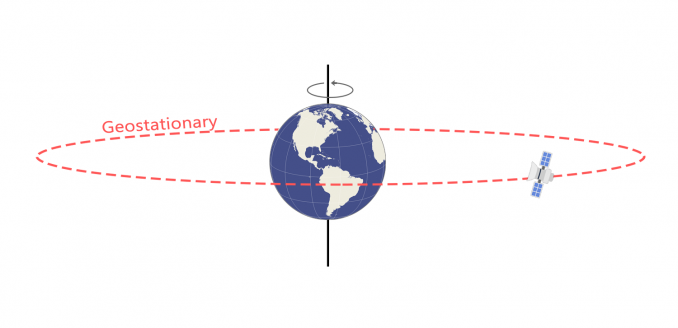Weather monitoring satellites like GOES are in geostationary orbits because they have a constant view of the same area. In a high Earth orbit, it’s also useful for search and rescue beacons.

Here’s how both orbits compare: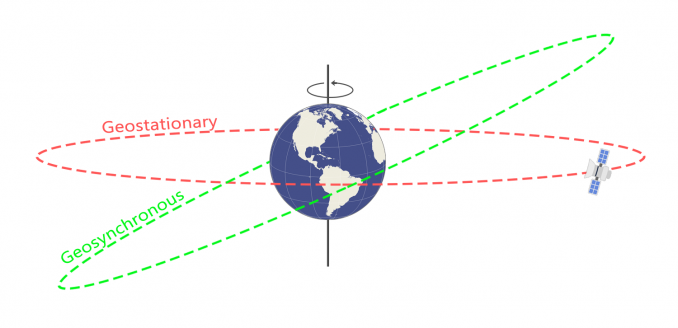While the geostationary orbit lies on the same plane as the equator, the geosynchronous satellites have a different inclination.

This is the key difference between the two types of orbits.

#### Semi-Synchronous Orbit

Global Positioning System (GPS) satellites are in another sweet spot known as semi-synchronous orbits. While geosynchronous orbits match the rotation of Earth (24 hours), semi-synchronous orbits take 12 hours to complete an orbit.

Instead of 35,786 kilometers above the Earth’s surface, semi-synchronous orbits are approximately 20,200 kilometers above the surface. This puts them in the medium Earth orbit range out of the three classes of orbits.

These orbits are close to zero in eccentricity, meaning they are near-circular. Eccentric orbits define how stretched orbits are. The closer eccentricity is to zero, the more the orbit closer to a circle. The closer to one, the orbit becomes longer and skinnier.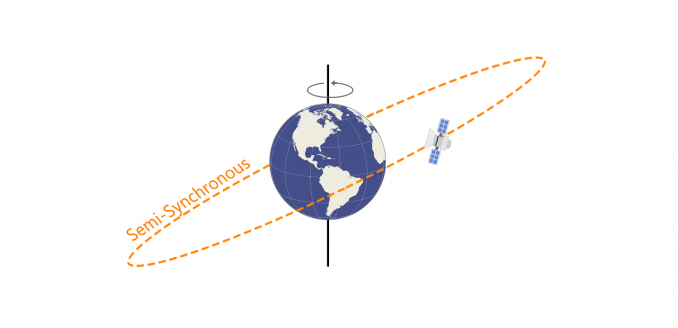For the 21st century and beyond, satellites will play an important role in some of the fundamental challenges.

Not to mention, the physics of satellite orbits are remarkable. And they have many practical purposes for science and innovation.

If you want to build on your expertise in satellite orbits, here a few more resources to expand your knowledge

1. Yared says:

Where is located the geosynchronous reference or coordinates or which real earth time calander?

2. Peter says:

I feel Pradeep Datta answer is incorrect as well. From an observers eye, there is NO possible way anyone could see a satellite the size of a small sedan.

3. RunawayPancake says:

Pradeep Datta’s comment above is correct with respect to inclined geosynchronous orbits. The article states that objects in a geosynchronous orbit don’t change position in the sky when viewed from Earth. This is true only if the object is in a geostationary orbit – a type of geosynchronous orbit that has 0° inclination with respect to the Equator. If the object is in a geosynchronouss orbit that is inclined with respect to the Equator, then the object will move in a figure eight pattern (aka an analemma) during the course of a sidereal day.

4. Bill S says:

I agree with G this would be better represented if the earth were shown at its 23.5 deg angle.

I feel, the explanation above is slightly incorrect, or at least a bit confusing.

In case of Geosynchronous Orbit you wrote
“If you are an observer on the ground, you would see the satellite as if it’s in a fixed position without movement.”

This is not correct. in such an orbit, it is ONLY synchronous to the sidereal day (23 hours, 56 minutes, and 4 seconds), meaning the satellite will be at the same position after this time. The orbit may have an inclination (to the equator) or even eccentricity. For an observer on the ground, the satellite shall describe a figure of eight. If the inclination to the equator is equal to zero and eccentricity is also zero, it becomes the special case of being Geostationary Orbit and the figure of eight being described by it to an observer on the ground becomes small being equal to zero, such that it now and only now appears to be stationary in the sky.

6. ANIL says:

Very good explanation

7. G says:

Nice explanation but I would consider showing the earth at its 23.5 deg angle.
Also, GPS satellites are at a 55 degree angle to the equator.

8. Willian Buarque says:

Really simple explanation!!!